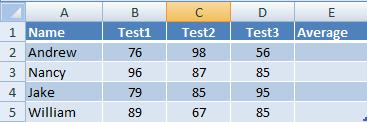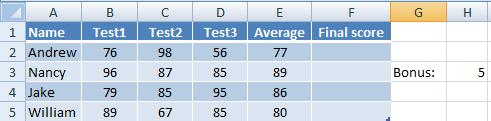Hide

# Absolute Referencing and Relative Referencing

You can download here the worksheet from this video to practice it by yourself!

Okay, this is usually the most dreaded and feared subject for students learning an Excel course.

Start by watching the video above. As with most of the videos in these tutorials, it explains this subject in the most simple, step-by-step manner. I'm sure the concept will be much clearer after you watch it.

In general, absolute and relative reference deals with what happens when you copy a formula to another cell.

The default action is that when you paste a formula, the addresses of cells inside it will change in accordance to the direction of where you pasted it. This is called relative reference.
For example, if you pasted one cell downwards, the numbers of the addresses inside it will increase by one.

There are occasions when you don't want the addresses inside a formula to change when you paste it. In this cases you will use absolute reference.
Let's examine closely the two situations.

## Relative Referencing

The formula’s default behavior in Excel is relative referencing.

Let’s understand this concept:
Assume that inside cell B5 you have the following formula:
=G2+1

If you copy this formula two cells downwards into cell B7 (either by doing “copy” and “paste”, or by dragging the fill handle), the formula pasted in B7 will be:
=G4+1

As you can see, copying a formula downwards, will change the address inside the formula, in accordance to how far you pasted from the original cell. If you pasted it two cells downwards (from B5 to B7), then the address inside the formula will change accordingly from G2 to G4.
If you pasted it one cell above the original (into cell B4) then the formula will be:
=G1+1

Similarly, if you pasted the formula in cell C5 (one cell to the right of the original cell), then the pasted formula will be:
=H2+1

Hence, pasting in a horizontal distance from the original cell, will cause a matching change in the column of the address inside the pasted formula.

When is this relative referencing useful?
Let’s assume you have 200 students, in a table like the following:Their average score (in column E) should be calculated using a formula.

The first formula in cell E2 can be:
=(B2+C2+D2)/3

(If you know functions, you can of course use the function: =average(B2:D2) which will give the same result)

This formula can be simply dragged downwards by the fill handle, and you will get in cell E3 the following formula:
=(B3+C3+D3)/3

And in cell E4 the following formula:
=(B4+C4+D4)/3

And that’s exactly what you need. And if you really had 200 students, all you need is to drag the formula 200 rows downwards, and you get 200 formulas, each one correctly corresponds to its own row.

## Absolute Referencing

Look at the following formula:
=\$G\$4+1

It’s actually the same formula as =G4+1, but with \$ signs.
The \$ signs might look a bit annoying to the eyes, making the formula less readable, but they have only one meaning to Excel: “Don’t change the address of cell G4 inside the formula when copied”. That’s why it’s called “Absolute Referencing”.

If you drag this formula downwards with the fill handle, you will get this:

=\$G\$4+1
=\$G\$4+1
=\$G\$4+1
=\$G\$4+1

and so on...
It doesn't change!

If you drag the following formula downwards: =B4*\$D\$5+A1
You will get:

=B5*\$D\$5+A2
=B6*\$D\$5+A3
=B7*\$D\$5+A4
=B8*\$D\$5+A5

How do you get these \$ signs?
When you type a formula, right after clicking a cell (hence getting its address inside the formula), push the F4 key in the keyboard.
You can also add these signs manually inside an address in a formula, by pressing the [Shift]+ keys at the correct places.

### When is absolute referencing useful?

Let’s continue with our previous student's scores example.
We now want to add another column (column F) which will represent a "final score". The final score is their average score, plus a bonus.
The bonus will be written in cell H3, as seen here:The final score (in column F) will be the average plus the bonus. So the formula in F2 might be:
=E2+H3

In words: the value of cell E2 (the average) plus the value of cell H3 (the bonus).

The formulas we need in the following cells of column F are:

=E3+H3
=E4+H3
=E5+H3

And so on...

As you see, the cell H3 should stay fixed. This will be achieved by typing the following formula (inside cell F2):

=E2+\$H\$3

Now we can drag this downwards with the fill handle and get the following correct formulas:

=E3+\$H\$3
=E4+\$H\$3
=E5+\$H\$3

### Conclusion:

Whenever you have a single specific cell that is relevant to many other cells, (you add it, or multiply it etc.), then this cell should be kept fixed in a formula by adding the \$ signs.

### Examples for cells that should stay fixed inside formulas:

A cell in your Excel worksheet that represents the tax in a specific region.
A cell that represents a discount percentage, or a bonus.
A cell that represents the currency exchange rate.
A cell that represents an interest rate.